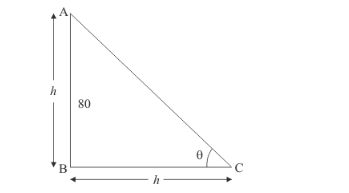# Find the angle of elevation of the sum (sun's altitude) when the length of

Question:

Find the angle of elevation of the sum (sun's altitude) when the length of the shadow of a vertical pole is equal to its height.

Solution:

Letbe the angle of elevation of sun. Letbe the vertical pole of heightandbe the shadow of equal length.

Here we have to find angle of elevation of sun.

We have the corresponding figure as followsSo we use trigonometric ratios to find the required angle.

In a triangle,

$\Rightarrow \quad \tan \theta=\frac{A B}{B C}$

$\Rightarrow \quad \tan \theta=\frac{h}{h}$

$\Rightarrow \quad \tan \theta=1$

$\Rightarrow \quad \theta=45^{\circ}$

Hence the angle of elevation of sun is $45^{\circ}$.